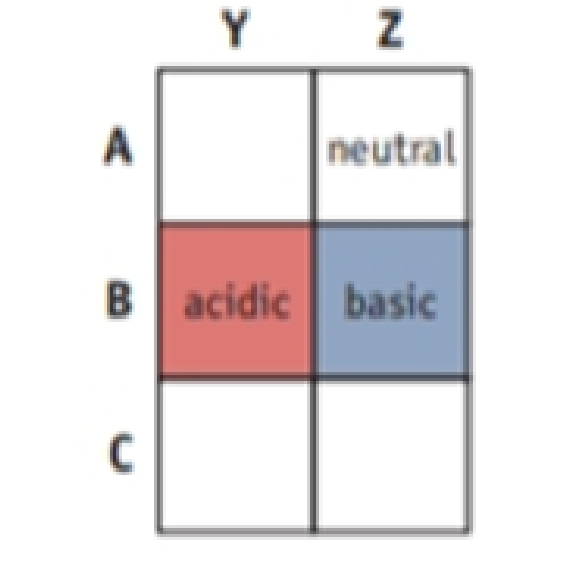# You have three solutions labeled A, B, and C. You know only that each contains a different cation—Na + , NH 4 + , or H 3 O + . Each has an anion that does not contribute to the solution pH (e.g., Cl − ). You also have two other solutions, Y and Z, each containing a different anion, Cl − or OH − , with a cation that does not influence solution pH (e.g., K + ). If equal amounts of B and Y are mixed, the result is an acidic solution. Mixing A and Z gives a neutral solution, whereas B and Z give a basic solution. Identify the five unknown solutions. (Adapted from D. H. Barouch: Voyages in Conceptual Chemistry , Boston, Jones and Bartlett, 1997.)### Chemistry & Chemical Reactivity

9th Edition
John C. Kotz + 3 others
Publisher: Cengage Learning
ISBN: 9781133949640

#### Solutions

Chapter
Section### Chemistry & Chemical Reactivity

9th Edition
John C. Kotz + 3 others
Publisher: Cengage Learning
ISBN: 9781133949640
Chapter 16, Problem 111IL
Textbook Problem
3 views

## You have three solutions labeled A, B, and C. You know only that each contains a different cation—Na+, NH4+, or H3O+. Each has an anion that does not contribute to the solution pH (e.g., Cl−). You also have two other solutions, Y and Z, each containing a different anion, Cl− or OH−, with a cation that does not influence solution pH (e.g., K+). If equal amounts of B and Y are mixed, the result is an acidic solution. Mixing A and Z gives a neutral solution, whereas B and Z give a basic solution. Identify the five unknown solutions. (Adapted from D. H. Barouch: Voyages in Conceptual Chemistry, Boston, Jones and Bartlett, 1997.)Interpretation Introduction

Interpretation:

Among three solutions A, B, and C each contain a different cation and the other two solutions Y and Z each containing a different anion. Five unknown solutions are to be stated.

Concept introduction:

Metal cations have two characteristics that allow them to act as a Lewis acid:

(a) Their positive charge that attracts electrons.

(b) They have at least one empty orbital that can accommodate an electron pair.

Anions can act as a Lewis base because they have a lone pair of the electron that can donate to an empty orbital of Lewis acid and anion can also act as a bronsted base because they can accept a proton from an acid to form the conjugate acid of the ion.

A conjugate acid-base pair contains two compounds that differ only by a hydrogen ion and a charge of +1.

The stronger the acid, the weaker its conjugate base and vice-verca.

### Explanation of Solution

Three solutions A, B, and C each containing different cations, Na+,NH4+ and H+ are

Lewis acid and two another solution Y and Z each containing different anions Cl,OH are Lewis bases.

A neutralization reaction can be defined as the reaction of an acid and base to produce salt and water. When a solution is neutralized it means that salt are formed from an equal amount of an acid and base. Depending on the acid-base properties of its ions, a salt can dissolve in water to produce a neutral solution, a basic solution, or an acidic solution.

There are three types of neutralization reaction as follows:

Strong Acid+StrongBaseSALT+H2O     pH=7(neutral)Strong Acid+WeakBaseSALT+H2O       pH<7(acidic)Weak Acid+StrongBaseSALT+H2O       pH>7(basic)

The Na+ cation is a Lewis acid, so it can combine with both Cl and OH ion. When a salt NaCl dissolves in water, it gives HCl and NaOH the reaction can be written as:

Na+(aq)+Cl(aq)+H2O(l)Na+(aq)+Cl(

### Still sussing out bartleby?

Check out a sample textbook solution.

See a sample solution

#### The Solution to Your Study Problems

Bartleby provides explanations to thousands of textbook problems written by our experts, many with advanced degrees!

Get Started

Find more solutions based on key concepts
What are the moral implications of using ergogenic aids?

Understanding Nutrition (MindTap Course List)

Type 2 diabetes often improves with a diet that is a. low in carbohydrates (less than 130 g per day). b. as low...

Nutrition: Concepts and Controversies - Standalone book (MindTap Course List)

Provide two reasons why meiosis leads to genetic variation in diploid organisms.

Human Heredity: Principles and Issues (MindTap Course List)

Identify and describe the tissues shown below.

Human Biology (MindTap Course List)

What type of metal associates with hemoglobin?

BIOLOGY:CONCEPTS+APPL.(LOOSELEAF)

Even a small loss of body weight can improve OA.

Nutrition Through The Life Cycle

Assume a sample of an ideal gas is at room temperature. What action will necessarily make the entropy of the sa...

Physics for Scientists and Engineers, Technology Update (No access codes included)